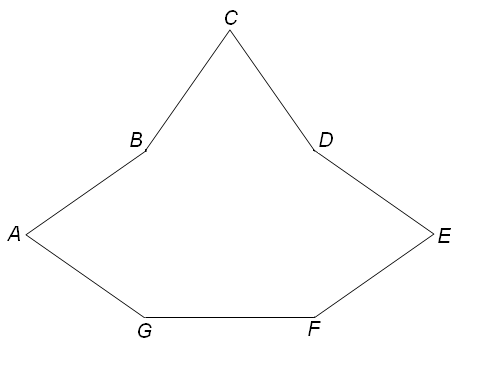#### You may also like### Building Tetrahedra

Can you make a tetrahedron whose faces all have the same perimeter?### Ladder and Cube

A 1 metre cube has one face on the ground and one face against a wall. A 4 metre ladder leans against the wall and just touches the cube. How high is the top of the ladder above the ground?Four rods are hinged at their ends to form a convex quadrilateral. Investigate the different shapes that the quadrilateral can take. Be patient this problem may be slow to load.

# Unusual Polygon

##### Age 14 to 16 Short Challenge Level:

The diagram shows a polygon ABCDEFG, in which $FG =6$ and $GA=AB=BC=CD=DE=EF$.

Also $BDFG$ is a square.

The area of the whole polygon is exactly twice the area of $BDFG$.

Find the length of the perimeter of the polygon.If you liked this problem, here is an NRICH task which challenges you to use similar mathematical ideas.

This problem is taken from the UKMT Mathematical Challenges.
You can find more short problems, arranged by curriculum topic, in our short problems collection.